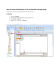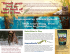# Outline R Crash Course

## Transcription

Outline R Crash Course
```Outline
R Crash Course
Introduction
Part 1: Working with Data
How to represent data?
Christophe {Lalanne, Pallier}
A simple tossing experiment
http://pallier.org/cours/stats.2011/
From vectors to matrix and dataframe
Cogmaster 2011
Import/export facilities
1 / 50
What is R?
2 / 50
How to interact with R?
You will need a “decent” text editor during this course.
Let’s not kid ourselves: the most widely used piece
of software for statistics is Excel.
—Brian Ripley
What do we expect from it?
Basically, we want good syntax highlight support, to send
command to an R process; an internal help display system is a
plus.
R is a statistical programming language for computing with
data.
Why R?
Not just because it is free and available on all platforms, but
because it is developed by an active community of statisticians
and end-users enclined to reropducible research. This means
good on-line help (mailing-list, wiki, package maintainer, the
StackExchange community), continuously updated packages,
top-notch algorithms, etc.
The general idea will be to write R code, test it in the console,
and keep related parts of our statistical progress in dedicated
R files. The penultimate idea is to learn to manage a
statistical project. See How to efficiently manage a statistical
3 / 50
Emacs + ESS
4 / 50
RStudio
http://ess.r-project.org/
http://rstudio.org/
5 / 50
6 / 50
• Tutorials, textbooks, etc.: See the CRAN docs section,
The core R packages might be sufficient in almost all the case
study we will encounter. Some recommend add-ons are listed
below:
http://cran.r-project.org/other-docs.html
• Getting help specific to R programming: R mailing,
http://stackoverflow.com
• Hmisc, a large set of utilities for importing/formatting
• Getting help specific to stats (language agnostic):
data, power analysis, clustering, tabular output.
• rms, everything we ever need for regression models.
• plyr, for data manipulation (reshaping, aggregating,
etc.).
• ggplot2, a high-level visualization backend.
http://crossvalidated.com
To install and update packages, the commands are:
install.packages and update.packages (default to CRAN,
but take a look at http://r-forge.r-project.org/).
7 / 50
Aims and scope of this course
8 / 50
Let’s get started
The objective is to teach you how to do statistical analysis on
your own. This means knowing a little bit of the R language
itself, as well as having a good understanding of statistical
theory and common techniques of data analysis. You’ll need to
practice a lot!
This session is all about the R language, but with some
incursion into statistical concepts like sampling distribution,
likelihood theory, parameter estimation.
9 / 50
R knows basic arithmetic
10 / 50
We can use R as a “simple” calculator.
Imagine a simple experiment, one consisting in tossing a coin.
> seq(1, 9, by=2)^2
> x <- c(0,1,1,0,1,0,1,0,0)
> x

1
9 25 49 81
 1
> x <- seq(-2, 2)
> t(x) %*% x * 1/(length(x)-1)
[1,]
> x[length(x)]
[,1]
2.5
 0
> x[2:4]
> rm(x)
> x <- sample(1:10, 20, replace=TRUE)
> x[ceiling(length(x)/2)]
 1 1 0
> x[c(2,4)]
 6
 1 0
> x[seq(1, length(x), by=2)]
 0 1 1 1 0
11 / 50
12 / 50
R vectors
More on addressing vector elements 1
Events were stored in a vector that consitutes the base R
patterns. What about their internal properties?
There are three fundamental types: numeric, character, and
logical. Consider the following “named” vector, x:
>
>
>
>
> is.vector(x)
 TRUE
> is.numeric(x)
x <- seq(1, 6)
names(x) <- letters[1:6]
y <- rep(c(T,F), 3)
x[x == 2] == x["b"]
 TRUE
b
TRUE
> cat(class(x), typeof(x), "\n")
> x[y]
numeric double
a c e
1 3 5
> x <- c(0L,1L,1L,0L,1L,0L,1L,0L,0L)
> cat(class(x), typeof(x), "\n")
> which(x %in% y)
integer integer
 1
13 / 50
More on addressing vector elements 2
Missing values
We’ll see more dictionnary-like facilities in the sequel of this
tutorial.
We can use boolean operators too.
Some data may be missing. R use NA to denote them.
> xc <- x
> xc[sample(1:6, 2)] <- NA
> is.na(xc)
> z1 <- sample(c(T,F), 6, replace=TRUE)
> z2 <- sample(c(T,F), 6, replace=TRUE)
> z2

TRUE
TRUE
TRUE FALSE
TRUE
a
TRUE
TRUE
b
c
d
e
f
TRUE FALSE FALSE FALSE FALSE
> which(is.na(xc))
> z1 & z2
 FALSE FALSE
14 / 50
a b
1 2
TRUE FALSE FALSE FALSE
> xc[is.na(xc)] <- "."
> print(xc)
> x[x < 5 & z2]
a b c
1 2 3
a
b
c
d
e
f
"." "." "3" "4" "5" "6"
15 / 50
Sorting
16 / 50
It is also possible to sort vectors, and work with ordered
indexes.
A better way to simulate this process might be
> x <- sample(c(0,1), 100, rep=T)
> table(x)
> xs <- sample(x)
> sort(xs, decreasing=TRUE)
f e d c b a
6 5 4 3 2 1
x
0 1
48 52
> xs[order(xs)]
a b c d e f
1 2 3 4 5 6
 "T" "T" "H" "T" "T" "T"
> rank(rev(xs))
 52
b f c a e d
2 6 3 1 5 4
Is there another way to generate such artificial data?
> sum(as.logical(x))
17 / 50
18 / 50
Probability distribution 1
Probability distribution 2
We could use a very simple probability law, namely the
uniform distribution (“all outcomes equally probable”) on the
[0, 1] interval and filter those results that are above or below
its centre point.
Here’s a possible solution:
Lookup the on-line R help:
>
>
>
>
> x <- runif(100)
> print(length(x[x < .5]))
 52
> apropos("unif")
> help.search("unif")
> if (require(sos)) findFn("unif")
# do it again, we'll get a different result
x <- runif(100)
xb <- ifelse(x < .5, 0, 1)
table(xb)
xb
0 1
57 43
19 / 50
Probability distribution 3
20 / 50
The tossing experiment revisited 1
But, we know that such events each follow a Bernoulli
distribution, and provided the trials are independent the
expected number of tails will follow a Binomial distribution, see
Tossing a coin
Failure
Success
5
●
●
●
●
●
●
●
●
●
●
6/10
4
●
●
●
●
●
●
●
●
●
●
7/10
3
●
●
●
●
●
●
●
●
●
●
5/10
2
●
●
●
●
●
●
●
●
●
●
6/10
1
●
●
●
●
●
●
●
●
●
●
3/10
1
2
3
4
5
6
7
8
9
10
> help(rbinom)
Sequence
Now, we can rewrite our little experiment.
>
>
>
+
>
>
n <- seq(10, 100, by=10)
res <- numeric(length(n))
for (i in seq_along(n))
res[i] <- table(rbinom(n[i], 1, .5))["1"]
names(res) <- n
print(round(res/n, 2))
10
20
30
40
50
60
70
80
90 100
0.60 0.55 0.43 0.42 0.54 0.40 0.50 0.46 0.43 0.46
Trial
21 / 50
The tossing experiment revisited 2
22 / 50
The tossing experiment revisited 3
0.20
Here are two theoretical distributions:
0.15
Please note that these are the results for each single run. Do
those results allow to draw a reliable conclusion about the
0.00
0.00
0.05
0.05
0.10
Density
0.10
0.15
again? How about running it 100 times and calculating the
average number of tails?
5
10
No. success
15
20
5
10
No. success
15
20
You tossed a coin 20 times; you got 13 success. Would you
say this is a fair coin? Are those results likely to come from
one of the above distribution?
23 / 50
24 / 50
Grouping instructions together 1
Grouping instructions together 2
How about reusing the same code several times? It would be
easier to call a single command with varying arguments. For
that, we need to write a function.
Here is a function for the tossing experiment:
We’ve already used a small simulation. Let’s look at the
following illustration of Marsaglia’s polar method:
>
>
>
+
+
+
+
+
+
+
>
n <- 500
v <- w <- z <- numeric(n)
for (i in 1:n) {
t <- runif(1)
u <- runif(1)
v[i] <- 2 * t - 1
w[i] <- 2 * u - 1
a <- v[i]^2 + w[i]^2
z[i] <- ifelse(a <=1 , a, NA)
}
round(summary(z), 2)
Min. 1st Qu.
0.00
0.23
Median
0.46
Mean 3rd Qu.
0.49
0.74
> get.tails <- function(n=5, p=0.5) sum(rbinom(n, 1, p))
with some examples of use:
Max.
1.00
>
>
>
>
>
>
>
NA's
111.00
get.tails()
get.tails(20)
get.tails(20, 0.75)
get.tails(p=0.75)
replicate(10, get.tails(n=100))
sapply(10:20, get.tails)
as.numeric(Map("get.tails", n=10:20))
26 / 50
25 / 50
Some of R RNGs
Below are some probability laws that we will use throughout
the course. Their parameters are reported in the first column
on the left.
A function in R is composed of a list of arguments (with or
without default value) and a body, which is a series of R
instructions. Usually, a value is returned, either the last
statement or the one enclosed in a call to return().
Here is an example (what does the function do actually
doesn’t matter):
> odds.ratio <- function(x, alpha=.05) {
+
if (!is.table(x)) x <- as.table(x)
+
pow <- function(x, a=-1) x^a
+
or <- (x[1,1] * x[2,2]) / (x[1,2] * x[2,1])
+
or.se <- sqrt(sum(pow(x)))
+
or.ci <- exp(log(or) + c(-1,1) * qnorm(1-alpha/2) * or.se)
+
return(list(OR=or, SE=or.se, CI=or.ci))
+ }
Binomial B(n, p)
rbinom pbinom
Uniform U[a,b]
runif
punif
Gaussian N (µ; σ 2 ) rnorm
pnorm
Student T (ν)
rt
pt
Chi square χ2 (ν)
rchisq pchisq
qbinom dbinom
qunif
dunif
qnorm
dnorm
qt
dt
qchisq dchisq
27 / 50
1
2
3
4
28 / 50
Recap’
Convert the Marsaglia’s code to a function (and simplify
the code, when possible).
Using the code related to the tossing experiment, let’s
take n = 50 and generate 100 experiments, store the
results, calculate the mean and variance.
Simulate a Binomial experiment (0/1), and lookup the
index of the first “one”. Using the same n and p, reiterate
a large number of times, say 1,000; check whether the
variance is approximately 1−p
.
p2
Simulate data from a Poisson process with a large n and
λ < 0.1. Compare to what you would get using a
Binomial distribution of parameter λ/n.
You should now be familiar with
• How to create and manipulate vectors, how to access
their elements.
• How to access the help system.
• How to generate random draws from a discrete or
continuous distribution.
• How to run simple experiment with a for loop.
• How to write little functions.
29 / 50
30 / 50
Beyond vectors
Working with matrix
Sometimes we need more than a single vector to represent our
data. Then, we can rely on matrix.
All ways to address vector values works here.
> m <- matrix(1:16, nc=4)
> m[3,2]
> x1 <- rpois(5, 3.8)
> x2 <- rpois(5, 3.8)
> rbind(x1, x2)
 7
> m[,2]
[,1] [,2] [,3] [,4] [,5]
x1
3
6
6
3
2
x2
3
4
4
2
12
 5 6 7 8
> m[c(1,3), c(2,4)]
Or
[1,]
[2,]
> matrix(c(x1, x2), nrow=2, byrow=TRUE)
[1,]
[2,]
[,1] [,2] [,3] [,4] [,5]
3
6
6
3
2
3
4
4
2
12
[,1] [,2]
5
13
7
15
> dim(m)
# i.e., nrow(m) x ncol(m)
 4 4
32 / 50
31 / 50
Row- and colwise operations
Yet another famous distribution
We can generate gaussian variates (i.e., realizations from the
“normal distribution”) using rnorm(). Say we want to fill a
100 × 10 matrix owith such values (10 draws of 100
observations).
Matrix are interesting because we can apply a lot of operations
by row or column. For example,
> m <- matrix(runif(100), nc=10)
> round(apply(m, 1, mean), 2)
> res <- matrix(nr=100, nc=10)
> for (i in 1:10)
+
res[,i] <- rnorm(100, mean=12, sd=2)
 0.47 0.54 0.54 0.38 0.59 0.58 0.43 0.50 0.46 0.61
> apply(m, 2, function(x) round(mean(x), 2))
A more efficient way to generate those data is to use the
replicate() function.
 0.57 0.55 0.47 0.36 0.53 0.51 0.61 0.44 0.52 0.52
Matrix objects will only hold values of the same type (e.g., all
character or all numeric).
Compute the following five-number summary for each column:
min, max, 1st and 3rd quartile, median, mean. See
help(summary).
33 / 50
Dataframe
34 / 50
More on dataframes 1
Dataframe are matrix-like object (more exactly, they are list)
but can hold vectors of different types.
Accessing elements from a dataframe might be done like for a
matrix, e.g.
> d <- data.frame(x=1:2, y=4:1, g=rep(c("m","f"),2))
> dim(d)
> d[2,2]
 3
 4 3
We can also use named columns:
> str(d)
> d\$y
'data.frame': 4 obs. of 3 variables:
\$ x: int 1 2 1 2
\$ y: int 4 3 2 1
\$ g: Factor w/ 2 levels "f","m": 2 1 2 1
 4 3 2 1
> d\$g
 m
Levels: f m
They can be used to store results of an experiment according
to certain conditions, some outcomes together with subjects’
characteristics, etc.
There are special operations available for this kind of R object
like
> summary(d)
35 / 50
36 / 50
More on dataframes 2
x
Min.
:1.0
1st Qu.:1.0
Median :1.5
Mean
:1.5
3rd Qu.:2.0
Max.
:2.0
y
Min.
:1.00
1st Qu.:1.75
Median :2.50
Mean
:2.50
3rd Qu.:3.25
Max.
:4.00
Factors 1
g
f:2
m:2
Factors are categorical variables allowing to establish a
distinction between statistical units, experimental conditions,
etc. They induce a partition of the dataset.
> # class(d\$g)
> levels(d\$g)
 "f" "m"
This is known as the long-format representation. Compare to,
e.g.
> unique(d\$g)
 m f
Levels: f m
> m <- matrix(d\$y, nc=2)
> colnames(m) <- rev(levels(d\$g))
> rownames(m) <- unique(d\$x)
> relevel(d\$g, ref="m")
 m f m f
Levels: m f
There are many ways to generate factors:
38 / 50
37 / 50
Factors 2
Illustration
> print(f1 <- factor(rep(1:2, length=4)))
Here are two series of height measurements from two classes:
 1 2 1 2
Levels: 1 2
> d <- data.frame(height=rnorm(40, 170, 10),
+
class=sample(LETTERS[1:2], 40, rep=T))
> d\$height[sample(1:40,1)] <- 220
> print(f2a <- as.factor(rep(c("a1", "a2"), each=2)))
 a1 a1 a2 a2
Levels: a1 a2
220
●
210
Height (cm)
> print(f2b <- gl(2, 2, labels=c("a1","a2")))
 a1 a1 a2 a2
Levels: a1 a2
200
●
190
●
●
170
●
●
160
● ●
●
●
●
●
●
●
●
●
●
10
●
●
●
●
●
●
0
●
●
●
●
●
●
●
●
●
●
●
●
●
●
180
20
30
● ●
●
40
Subject
Does the taller come from class A or B? Which class shows
more variability with respect to height?
39 / 50
Internal datasets
40 / 50
Importing data 1
R comes with a lot of example datasets. They can be loaded
using the data() command. Some of them are directly
available from the base packages, others can be imported from
To list all available datasets, you can try:
R can read data from a variety of proprietary format (SPSS,
Stata, SAS), “connections”, but also simple tab-delimited or
csv file.
Here is an example with a tab-delimited dataset:
> data()
If you already know the name of the dataset, you can use it
directly, e.g.
1
2
3
> data(sleep)
> help(sleep)
> data(ISwR::intake)
stages
local
local
local
year n
1974 5
1975 8
1976 10
> table(colcan\$stages)
local regional
12
12
> with(colcan, tapply(n, stages, sum))
41 / 50
42 / 50
Importing data 2
Writing data 1
local regional
112
32
Sometimes, it might be interesting to save data or results to
disk. We can use quite the same commands, e.g.
write.table().
However, here are some possible alternatives to save results to
a file:
And here is an example with an Excel file converted to csv:
> str(perinatal)
'data.frame': 74 obs. of 5 variables:
> n <- 100
\$ weight : Factor w/ 37 levels "<800",">4300",..: 1 36 37 3 4 5 6 7 8 9 ... > d <- data.frame(age=sample(20:35, n, rep=T),
\$ infants: Factor w/ 2 levels "black","white": 1 1 1 1 1 1 1 1 1 1 ...
+
y=rnorm(n, 12, 2),
\$ deaths : int 533 65 40 30 29 21 19 19 20 24 ...
+
gender=gl(2, n/2, labels=c("male", "female")))
\$ births : int 618 131 122 131 137 143 143 165 167 219 ...
> cat("Mean of y is:", mean(d\$y), "\n", file="out1.txt")
\$ prob
: num 862 496 328 229 212 ...
Check your working directory with getwd(). Using save() and
load(), you can work with R objects saved in a binary image.
44 / 50
43 / 50
Writing data 2
Or,
1
> sink("out2.txt")
> with(d, tapply(y, gender, mean))
male
female
11.77841 11.92955
2
> sink()
3
Or,
> capture.output(summary(d), file="out3.txt")
4
To check whether those files were saved, you can use:
> list.files(dir(), pattern="*.txt")
5
The odds.ratio() function expects a 2 × 2 table or
matrix. Check that these conditions are fullfilled at the
beginning of the function body.
Write a summary-like function including the SD in
Load the sleep dataset and summarize the difference in
hours of sleep for both groups.
Load the USArrests dataset and summarize all numeric
variables. Reorder the dataset by numbr of murders.
Hint: See help(do.call).
Create a fake dataset comprised of two columns of
gaussian variates and a factor. Export it as a csv file.
45 / 50
Recap’
46 / 50
Some words of caution
Be careful with floating point arithmetic. (1,2)
You should now be familiar with
• How to work with matrix and dataframe objects.
> .1 == .3 / 3
• How R represents qualitative or (ordered) discrete
 FALSE
variables.
• How to avoid looping for row-, col-, and groupwise
computations.
• How to read external data files.
• How to write intermediate results to your HD.
> any(seq(0, 1, by=.1) == .3)
 FALSE
Factors are internally stored as numeric values and levels are
recorded in lexicographic order.
> as.numeric(f <- factor(1:2, levels=2:1))
 2
> as.numeric(levels(f))[f]
 1
47 / 50
48 / 50
References 1
List of R commands
1. P Burns. The R Inferno, 2011. URL http://www.burns-stat.com.
&, 15
any, 48
apply, 33
apropos, 21
c, 11
capture.output, 45
cat, 13
data, 41
data.frame, 35
factor, 39
function, 26
gl, 39, 45
help, 26
help.search, 21
ifelse, 21
2. D Goldberg. What every computer scientist should know about
floating-point arithmetic. ACM Computing Surveys, 23(1), 1991. URL
http://www.validlab.com/goldberg.
49 / 50
is.na, 16
length, 11
levels, 39
Map, 26
matrix, 31
mean, 33
names, 17
numeric, 26
order, 17
rank, 17
rbind, 31
rbinom, 26
relevel, 39
rep, 15
replicate, 34
rev, 17
rnorm, 34
round, 26
rpois, 31
runif, 21
sample, 11
sapply, 26
seq, 11
sink, 45
sort, 17
sum, 18
t, 11
table, 18
tapply, 43
unique, 39
Last updated: September 28, 2011. LATEX + pdfTEX and R 2.13.1.
50 / 50
```

### Grade 2 Unit 1 Patterns Identify, describe, extend, and create numeric patterns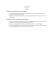### Kinkajou - Greensboro Science Center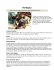### MAINE LOBSTER TAILS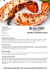### Discover 3DESIGN, the most powerful 3D jewelry solution available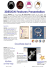### How to Export Distribution List from Outlook to Google Apps Contacts